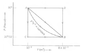# Callen Thermodynamics Problem

Eldorin

## Homework Statement

A particular gas is enclosed in a cylinder with a moveable piston. It is observed that if the walls are adiabatic, a quasi-static increase in volume results in a decrease in pressure according to the equation
a ) Find the quasi-static work done on the system and the net heat transfer to the system in each of the three processes (ADB, ACB, and the direct linear process AB ) as shown in the figure.
In the process ADB the gas is heated at constant pressure ( P = 10^5 Pa) until its volume increases from its initial value of 10^-3 m^3 to its final value of 8e-3 m^3. The gas is then cooled at constant volume until its pressure decreases to 10^5/32 Pa. The other processes (ACB and AB) can be similarly interpreted, according to the figure.

P^3*V^5 =Constant for Q=0

I am currently only having trouble with the part with the direct linear process AB.

dQ = δU - δW
W = -PdV

## The Attempt at a Solution

When it says it is directly linear, I took that to mean the following:

P*V = Px*Vx
Where Px and Vx are the initial pressure and volume. From there I integrated the work equation and got the following:

W = -Px*Vx*ln(Vb/Va).

I then did the same thing with the P^3*V^5 = Constant equation, but when I used these, as worked with the previous parts. My answers did not match the ones given in the book (W = - 360.9 J and Q = 248.4J)

I appreciate any help, thanks!

#### Attachments

•Screen shot 2013-08-22 at 4.12.09 PM.png
5.9 KB · Views: 758

Homework Helper
Gold Member
Hello, Eldorin. Welcome to PF!

"Linear process" means that P is a linear function of V so that the graph is a straight line on the PV diagram.

So, that means P = aV + b for certain constants a and b. However, you shouldn't need to use this equation since you can determine the work W graphically from the PV diagram.

Eldorin
Still having a bit of trouble

Alright, so I tried going through this and experienced some additional problems.

So, I'd like to know how to solve it both graphically and not graphically.

I linearized the equation, P=a*V+b, where P1=10^5, V1 = 10^-3, P2 = 10^5/32, V2 = 8e-3.

From this I determined that a = -1.384, b=10^5 (Both rounded).

I then set up dU = dW = -PdV, integrated and found that Ub-Ua = 0.5*(1.384*(Vb^2-Va^2))-(10^5(Vb-Va)) = -700J.

From there, I graphically found (What I now assume to be Q, since it gets me the correct answer later in the progression. Can anyone explain why this is, but the Q answer I get doesn't match the answer?) by solving for the area under the triangle and got Q=339.06J. From there I set up

Ub-Ua = Q - W, and solved for W and got -360.94J.

At this point, I'm pretty thoroughly confused. Can anyone explain what I did wrong, how to find the solution without using the graph, and what solving it graphically actually gives, since it appears to not be Q or W.

Thanks much!

Last edited:
Homework Helper
Gold Member
Your value of a is off by a certain power of 10. That makes your value of b incorrect.

When finding the work graphically, you need to find the area between the linear path and the V axis. This is the area of a trapezoid.

For the linear process, dQ is not zero.

If you already found ΔU for one of the other paths, does that help you find ΔU for the linear path? Remember, U is a state variable.

Eldorin
One more quick question so I can make sure I understand this completely. I've gotten the correct answer now, so it's no longer a problem with that, but more a conceptual problem.

Why are we allowed to assume that Q=0 for the P^3*V^5 = constant in order to simplify the equation from dU=dW+dQ to only dU=dW. Is this because we are assuming that it is a quasi-static work (i.e. done so slowly that none of the work is converted into waste heat) or is there another reason?

Thanks again for all of your help!

Homework Helper
Gold Member
Why are we allowed to assume that Q=0 for the P^3*V^5 = constant in order to simplify the equation from dU=dW+dQ to only dU=dW. Is this because we are assuming that it is a quasi-static work (i.e. done so slowly that none of the work is converted into waste heat) or is there another reason?

It is not because the work is quasi-static that Q equals zero. For example, in all three processes in your problem, you would assume the work is quasi-static so that the pressure is well-defined at each point of the process. Quasi-static just means that the process is slow enough that the system is essentially in thermal equilibrium at each point of the process. Essentially, any time you see a continuous path drawn on a PV diagram, you can assume that the process is quasi-static.

The reason that Q = 0 for the process in which P3V5 = constant is because that is what was given to be true in the statement of the problem. (The word "adiabatic" implies Q = 0.)